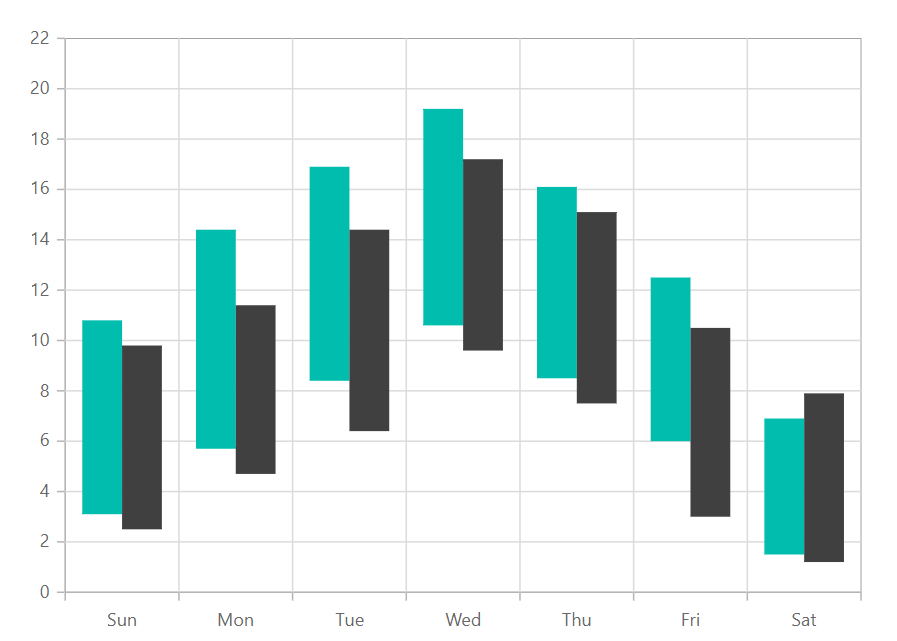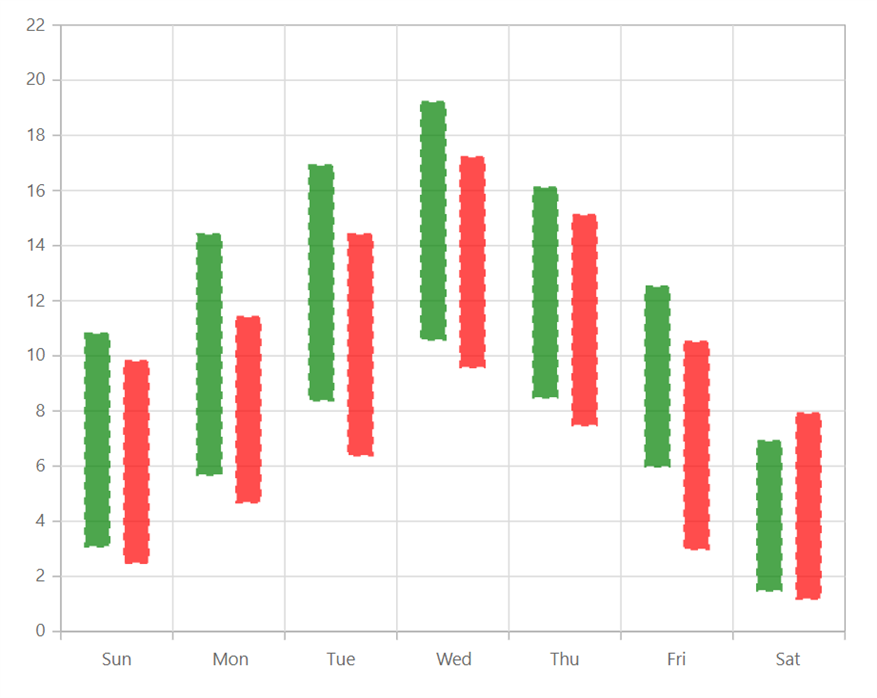Search results

# Range Column in Blazor Chart component

08 Apr 2021 / 3 minutes to read

## Range

Like the column chart, but shows the variations in the data values for a given time using vertical bars. To render a range column series, use series `Type` as `RangeColumn`.

rangecolumn.razor
``````@using Syncfusion.Blazor.Charts

<SfChart>
<ChartPrimaryXAxis ValueType="Syncfusion.Blazor.Charts.ValueType.Category"></ChartPrimaryXAxis>

<ChartSeriesCollection>
<ChartSeries DataSource="@WeatherReport1" XName="X" High="High" Low="Low" Width="2" Type="ChartSeriesType.RangeColumn">
</ChartSeries>
<ChartSeries DataSource="@WeatherReport2" XName="X" High="High" Low="Low" Width="2" Type="ChartSeriesType.RangeColumn">
</ChartSeries>
</ChartSeriesCollection>
</SfChart>

@code{
public class ChartData
{
public string X { get; set; }
public double Low { get; set; }
public double High { get; set; }
}
public List<ChartData> WeatherReport1 = new List<ChartData>
{
new ChartData { X= "Sun", Low= 3.1, High= 10.8 },
new ChartData { X= "Mon", Low= 5.7, High= 14.4 },
new ChartData { X= "Tue", Low= 8.4, High= 16.9 },
new ChartData { X= "Wed", Low= 10.6, High= 19.2 },
new ChartData { X= "Thu", Low= 8.5, High= 16.1 },
new ChartData { X= "Fri", Low= 6.0, High= 12.5 },
new ChartData { X= "Sat", Low= 1.5, High= 6.9 }
};

public List<ChartData> WeatherReport2 = new List<ChartData>
{
new ChartData { X= "Sun", Low= 2.5, High= 9.8 },
new ChartData { X= "Mon", Low= 4.7, High= 11.4 },
new ChartData { X= "Tue", Low= 6.4, High= 14.4 },
new ChartData { X= "Wed", Low= 9.6, High= 17.2 },
new ChartData { X= "Thu", Low= 7.5, High= 15.1 },
new ChartData { X= "Fri", Low= 3.0, High= 10.5 },
new ChartData { X= "Sat", Low= 1.2, High= 7.9 }
};
}``````Note: You can refer to our `Blazor Range Column Chart` feature tour page to know about its other groundbreaking feature representations. You can also explore our `Blazor Range Column Chart Example` to know how to show variations in the data values for a given time.

## Customization

You can use the following properties to customize the range column series.

``````@using Syncfusion.Blazor.Charts

@using Syncfusion.Blazor.Charts
<SfChart>
<ChartPrimaryXAxis ValueType="Syncfusion.Blazor.Charts.ValueType.Category"></ChartPrimaryXAxis>

<ChartSeriesCollection>
<ChartSeries DataSource="@WeatherReport1" XName="X" High="High" Low="Low" Width="2" Type="ChartSeriesType.RangeColumn" Fill="green" ColumnSpacing="0.2" Opacity="0.7" >
</ChartSeries>
<ChartSeries DataSource="@WeatherReport2" XName="X" High="High" Low="Low" Width="2" Type="ChartSeriesType.RangeColumn" Fill="red" ColumnSpacing="0.2" Opacity="0.7" >
</ChartSeries>
</ChartSeriesCollection>
</SfChart>

@code{
public class ChartData
{
public string X { get; set; }
public double Low { get; set; }
public double High { get; set; }
}
public List<ChartData> WeatherReport1 = new List<ChartData>
{
new ChartData { X= "Sun", Low= 3.1, High= 10.8 },
new ChartData { X= "Mon", Low= 5.7, High= 14.4 },
new ChartData { X= "Tue", Low= 8.4, High= 16.9 },
new ChartData { X= "Wed", Low= 10.6, High= 19.2 },
new ChartData { X= "Thu", Low= 8.5, High= 16.1 },
new ChartData { X= "Fri", Low= 6.0, High= 12.5 },
new ChartData { X= "Sat", Low= 1.5, High= 6.9 }
};

public List<ChartData> WeatherReport2 = new List<ChartData>
{
new ChartData { X= "Sun", Low= 2.5, High= 9.8 },
new ChartData { X= "Mon", Low= 4.7, High= 11.4 },
new ChartData { X= "Tue", Low= 6.4, High= 14.4 },
new ChartData { X= "Wed", Low= 9.6, High= 17.2 },
new ChartData { X= "Thu", Low= 7.5, High= 15.1 },
new ChartData { X= "Fri", Low= 3.0, High= 10.5 },
new ChartData { X= "Sat", Low= 1.2, High= 7.9 }
};
}``````Note: You can refer to our `Blazor Charts` feature tour page for its groundbreaking feature representations. You can also explore our `Blazor Chart example` to knows various chart types and how to represent time-dependent data, showing trends in data at equal intervals.

Contents
Contents